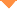首页 > 论文 > Chinese Optics Letters > 17卷 > 8期(pp：08)

The interactive length of fundamental wave and second harmonic generation generated on the surface of anomalous dispersion medium

• 摘要• 论文信息• 参考文献• 被引情况In this article, a new method is presented to calculate the interactive length between fundamental wave (FW) and second harmonic generation (SHG) for the configuration of total internal reflection on the inner surface of nonlinear crystal. Three independent experiments are designed to measure bandwidths of this second harmonic wave. The theoretical expression of the intensity of SHG is obtained through nonlinear coupled wave equation. By utilizing the measured bandwidths and the intensity equation through mathematical calculation, the interactive length of this phase-matched SHG can be calculated. Because there is no existing method to obtain the interactive length either from the theoretical calculation or experimental measurement. This method can be applied to estimate the extremely short interactive volume in nonlinear processes.

Institute of Super-microstructure and Ultrafast Process in Advanced Materials
Central South University

Wang Xiaojing,Ren Huaijin,Wang Gang,He Jun. The interactive length of fundamental wave and second harmonic generation generated on the surface of anomalous dispersion medium[J].Chinese Optics Letters,2019,17(8):08.